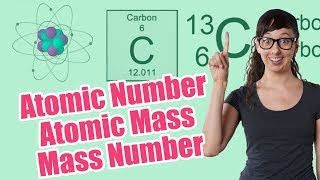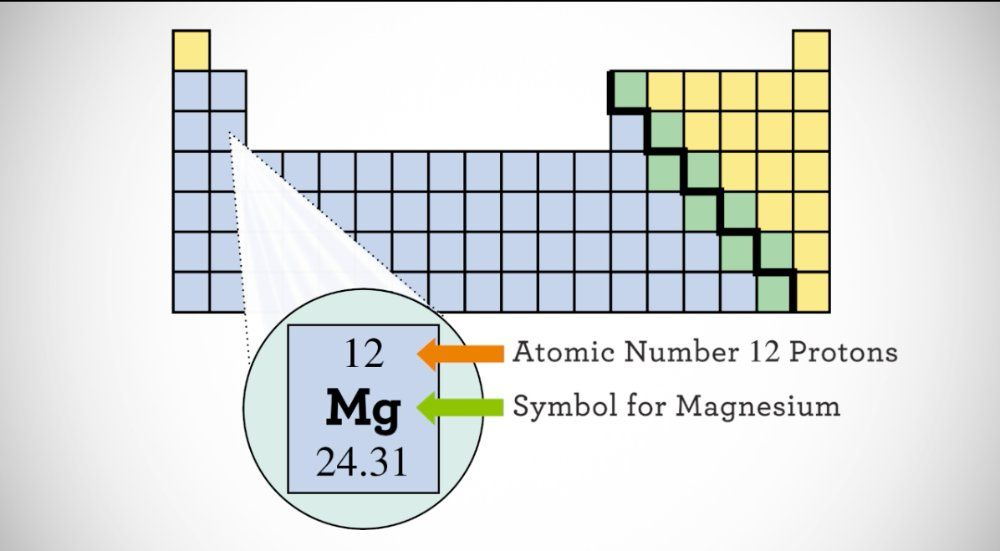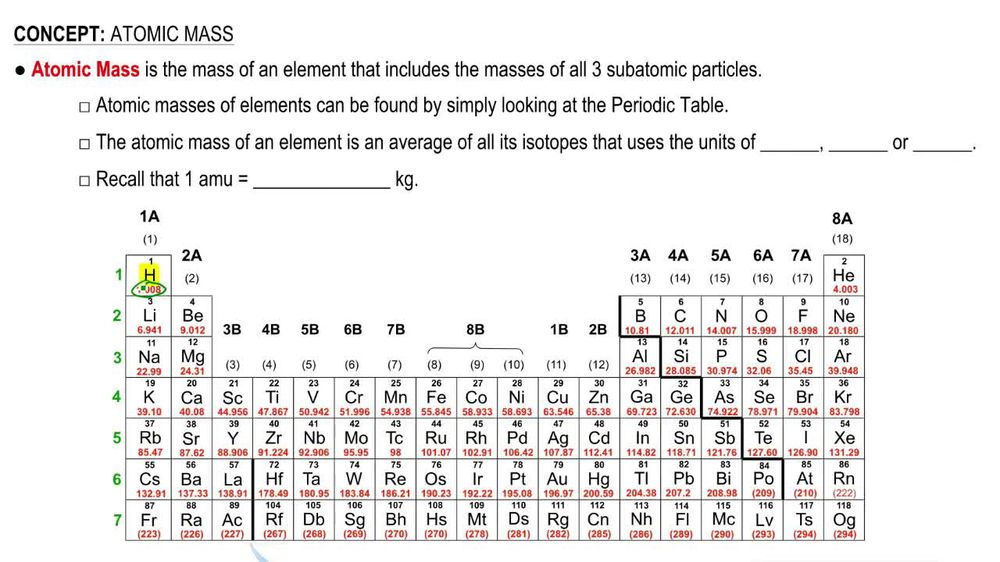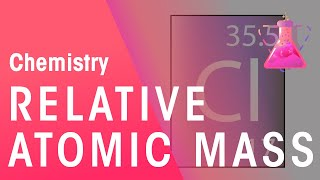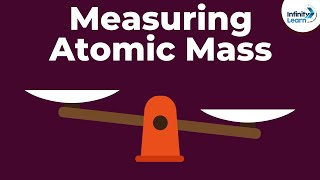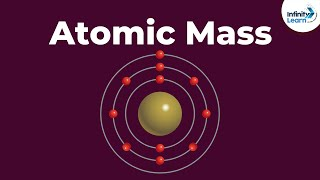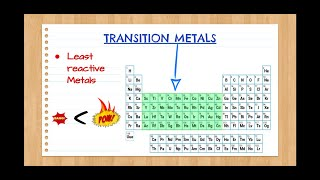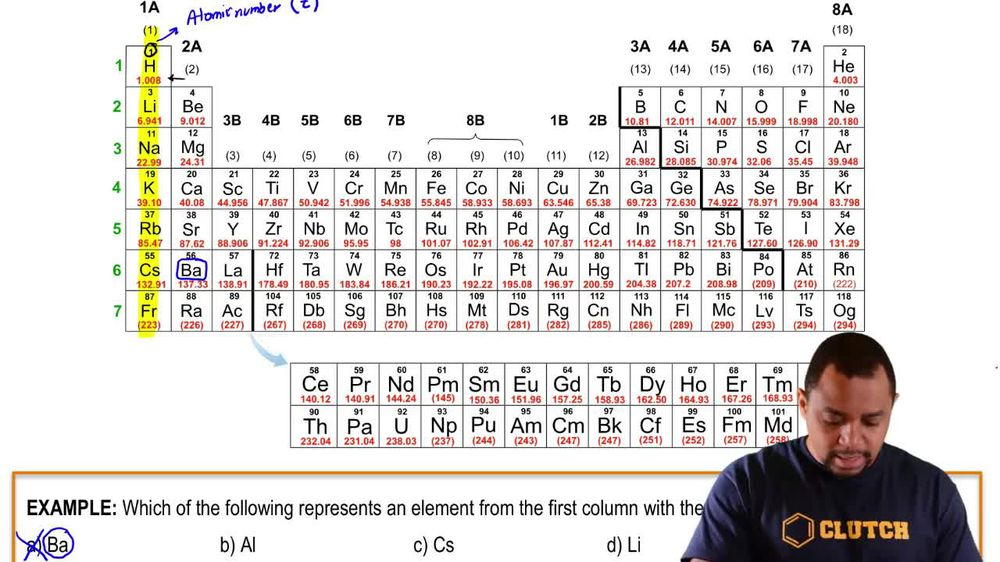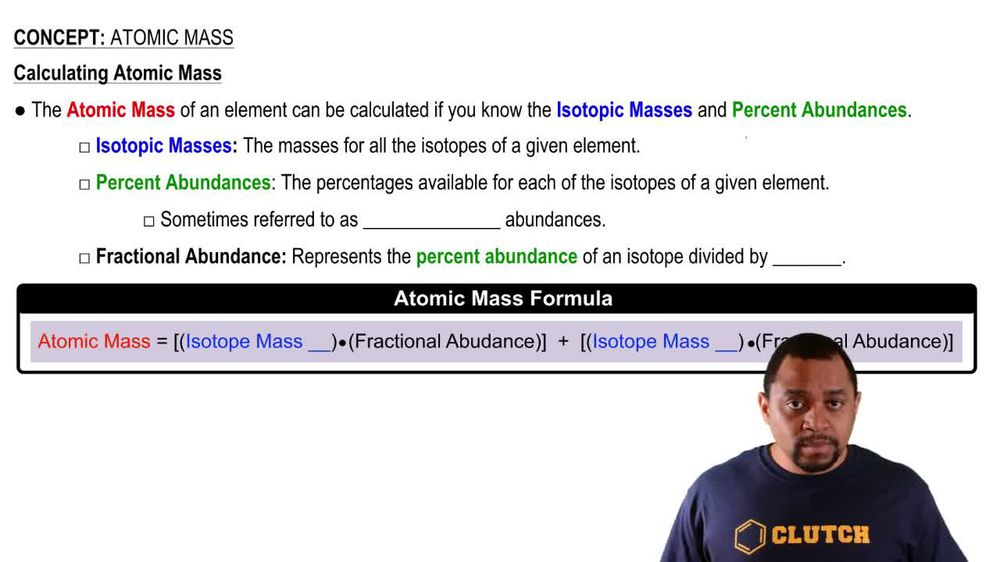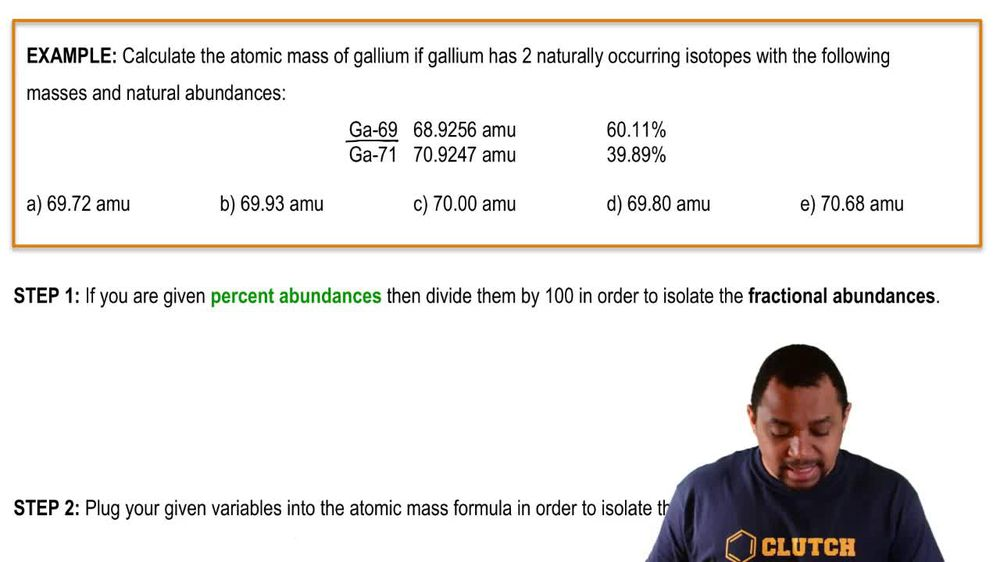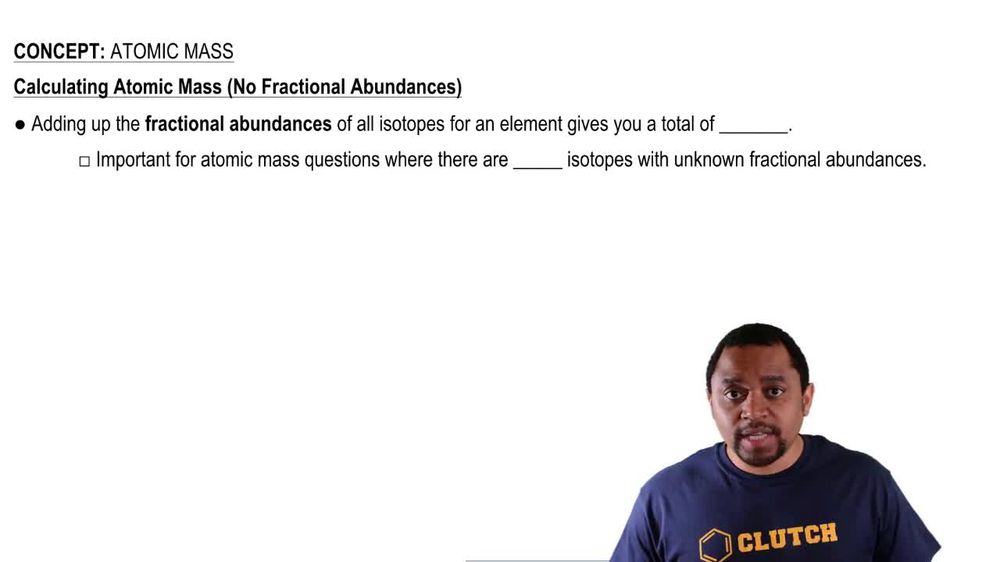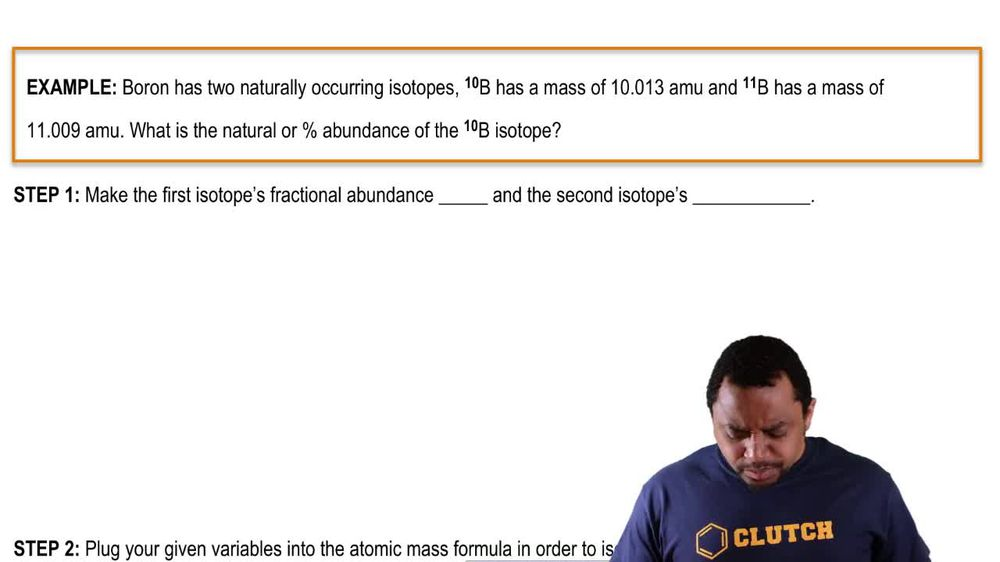Start typing, then use the up and down arrows to select an option from the list.
1. 2. Atoms & Elements2. Atomic Mass
Problem

# Naturally occurring silver consists of two isotopes: 107^Ag (51.84%) with an isotopic mass of 106.9051 and 109^Ag (48.16%) with an isotopic mass of 108.9048. What is the atomic weight of silver? Check your answer in a periodic table.

Relevant Solution2m
Play a video:
Hey everyone. So our question wants us to calculate the atomic weight of silver and were given some key information here, such as the fractional abundances of two naturally occurring isotopes and their masses for this question we're going to need to recall the following formula. So we have our atomic mass is going to be equal To the mass of one of our isotopes. So I'll label this as silver 107 and this is going to be an atomic mass units and we're going to multiply this by its fractional abundance, which I'll label as F A. We're also going to add the same exact thing But instead in terms of Silver 109, now that we have our formula, let's go ahead and plug in our values. So we have Silver 107 mass which is 106.9 I am you And we're going to multiply this by its fractional abundance of 51.82%. Well we're first going to convert that into its decimal form so that's going to be .5182. And we just moved our decimal places, two places to the left to convert our percentage into its decimal form. We're also going to add The mass of silver Which is 108 .9 a. m. you and we're going to multiply that by 48.18% in its decimal form. So calculating this out, we're going to end up with 55.396. I am u plus 52.468. I am you Adding these two values together? We end up with an atomic mass of 107 .9 a. m. you so this is going to be our final answer. So I hope that made sense and let us know if you have any questions.